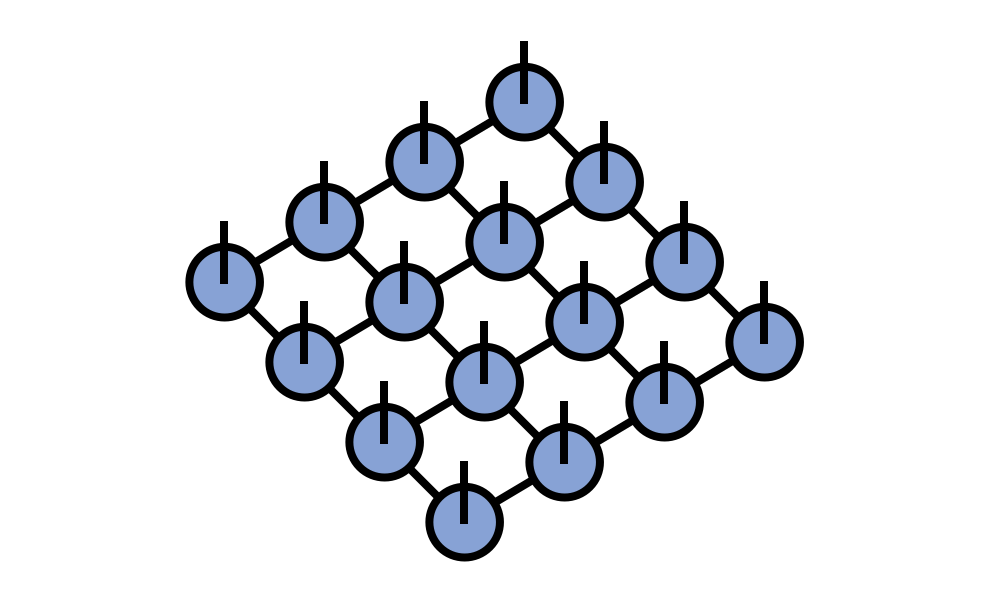About Contribute Source main/peps/

# PEPS Tensor Network

The PEPS tensor network (projected entangled pair states) generalizes the matrix product state / tensor train tensor network from a one-dimensional network to a network on an arbitrary graph.

The tensor diagram for a PEPS on a finite square lattice is:## Explanation of the Name PEPS

The name PEPS comes from a quantum information viewpoint that generic tensor networks can be viewed as maximally correlated (or entangled) tensors in multiple copies of the tensor index space which are then projected into a single copy of the index space.

In an more general mathematics context, a better name for these networks might be tensor grid or tensor lattice networks.

## Applications of PEPS in Quantum Physics

PEPS tensor networks have primarily been used as an ansatz for quantum wavefunctions, more specifically ground states of two-dimensional Hamiltonians.

## References

1. Renormalization algorithms for Quantum-Many Body Systems in two and higher dimensions, F. Verstraete, J. I. Cirac (2004), cond‑mat/0407066
2. Valence-bond states for quantum computation, F. Verstraete, J. I. Cirac, Phys. Rev. A 70, 060302 (2004), quant‑ph/0311130